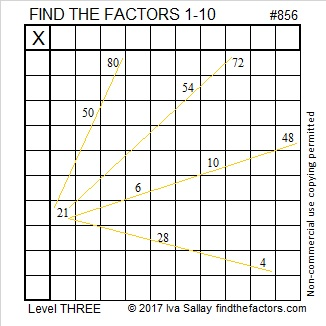# 856 Rays of Light

When we learn something new, it is as if rays of light touch our minds. If you know how to multiply and divide, let this puzzle enlighten your mind. Just start at the top of the first column and work down cell by cell until the numbers 1 to 10 have been written in the first column and the top row and those corresponding numbers multiply together to give the clues in the puzzle.Print the puzzles or type the solution on this excel file: 10-factors-853-863

• 856 is a composite number.
• Prime factorization: 856 = 2 × 2 × 2 × 107, which can be written 856 = 2³ × 107
• The exponents in the prime factorization are 3 and 1. Adding one to each and multiplying we get (3 + 1)(1 + 1) = 4 × 2 = 8. Therefore 856 has exactly 8 factors.
• Factors of 856: 1, 2, 4, 8, 107, 214, 428, 856
• Factor pairs: 856 = 1 × 856, 2 × 428, 4 × 214, or 8 × 107
• Taking the factor pair with the largest square number factor, we get √856 = (√4)(√214) = 2√214 ≈ 29.2574777